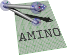amino  1.0-beta2 Lightweight Robot Utility Library
spatial.hpp File Reference

Object types for SE(3) representations. More...

Go to the source code of this file.

## Classes

struct  amino::Vec3
A vector of length 3. More...

struct  amino::XAngle
A rotation about the X axis. More...

struct  amino::YAngle
A rotation about the Y axis. More...

struct  amino::ZAngle
A rotation about the Z axis. More...

struct  amino::Quat
A quaternion object. More...

struct  amino::RotMat
A rotation matrix object. More...

struct  amino::AxisAngle
An axis-angle object. More...

struct  amino::DualQuat
A dual quaternion object. More...

struct  amino::QuatTran
A rotation quaternion and translation vector object. More...

struct  amino::TfMat
A transformation matrix object. More...

amino
amino namespace

## Functions

static struct aa_tf_vec3 amino::operator+ (const struct aa_tf_vec3 &a, const struct aa_tf_vec3 &b)

static struct aa_tf_vec3 amino::operator/ (const struct aa_tf_vec3 &a, double b)
Divide a Vec3 by a scalar.

static struct aa_tf_rotmat amino::operator* (const struct aa_tf_rotmat &a, const struct aa_tf_rotmat &b)
Multiply two rotation matrices.

static struct aa_tf_quat amino::operator* (const struct aa_tf_quat &a, const struct aa_tf_quat &b)
Multiply two ordinary quaternions.

static struct aa_tf_tfmat amino::operator* (const struct aa_tf_tfmat &a, const struct aa_tf_tfmat &b)
Multiply two transformation matrices.

static struct aa_tf_duqu amino::operator* (const struct aa_tf_duqu &a, const struct aa_tf_duqu &b)
Multiply two dual quaternions.

static struct aa_tf_qv amino::operator* (const struct aa_tf_qv &a, const struct aa_tf_qv &b)
Chain two poses stored in quaternion-vector form. More...

static struct aa_tf_vec3 amino::operator* (const struct aa_tf_rotmat &r, const struct aa_tf_vec3 &p)
Rotate point by rotation matrix.

## Detailed Description

Object types for SE(3) representations.

The class definitions in this file provide convenience methods to simplify type conversions between various orientation and transformation representations. The underlying operatations (and many others) are declared in tf.h.